# Stalgo

STALGO is an industrial-strength C++ software package for computing straight skeletons and mitered offset-curves, which I developed during my PhD studies. Its main features are:

• STALGO is efficient. For a dataset (e.g., a polygon) with n vertices it requires roughly 10 n log n microseconds and 2000 n bytes of main memory to compute the straight skeleton. In comparison to the straight-skeleton code that is shipped with the CGAL library, STALGO is by a linear factor faster and by a linear factor more space efficient. That is, STALGO typically uses two to three orders of magnitude less time and memory. (An up-to-date runtime comparison can be found in [HuHe12].)

• STALGO processes general input. STALGO not only takes polygons or polygons with holes as input but accepts any planar straight-line graphs as input, i.e., any arrangement of straight-line segments that do not intersect in their interior.

• STALGO computes mitered offset curves and roof models. Mitered offset curves and roof models can be easily computed from the straight skeleton in an efficient and reliable fashion.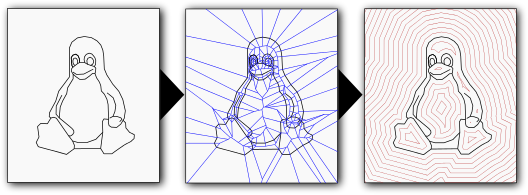To learn more about STALGO, straight skeletons and applications, you may visit my web page on our research conducted on straight skeletons.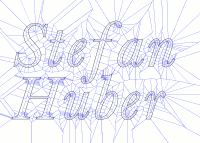The straight skeleton of the font outline of 'Stefan Huber'.Some offset curves of the left dataset.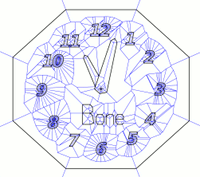A planar straight-line graph with vertices of degrees 1, 2, 3 and 4.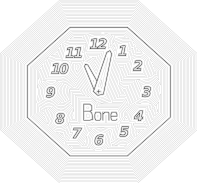Some offset curves of the left dataset.A tribute to the Linux operating system: the straight skeleton of its mascot, the Linux penguin Tux. Note that the input does not only consist of simple polygons.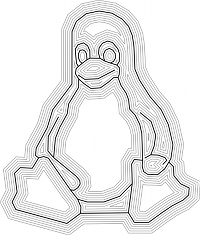Some offset curves of the left dataset.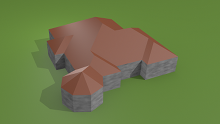A roof model generated by STALGO using the straight skeleton of the polygon formed by the walls. The rendering was done in Blender.A terrain model generated by STALGO. The present terrain illustrates the Schlögener Schlinge, a part of the Austrian river Danube. The river bank serves as input.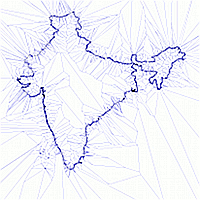A straight skeleton of a map of india, containing 16530 vertices.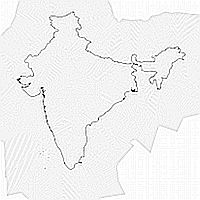Some offset curves of the left dataset.